# Euler product

An Euler product is a representation of an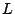-function as a convergent infinite product over the primes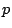, where each factor (called the local factor at'') is a Dirichlet series supported only at the powers of.

The Riemann $\zeta$-function has Euler product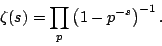A Dirichlet $L$-function has Euler product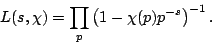The Dedekind zeta function of a number field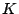has Euler product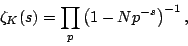where the product is over the prime ideals of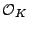.

An $L$-functions associated with a newform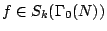or a Maass newform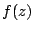on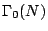has Euler product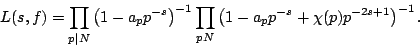$GL(r)$ $L$-functions have Euler products where almost all of the local factors are (reciprocals of) polynomials in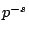of degree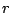.

Back to the main index for The Riemann Hypothesis.